# Hands-On: Unsupervised Learning - Clustering¶

### AI and Predictive Analytics in Data-Center Environments - http://dcai.bsc.es¶

In this hands-on we'll take a look to unsupervised learning through the quite simple yet powerful K-means algorithm. We will start by importing the required packages as usual:

In :
import numpy as np
import pandas as pd
import matplotlib.pyplot as plt


And setting a seed for repeatability:

In :
seed=25740565


For illustration purposes we will generate our own data for this example so we can have full control over it. Let's start by defining the cluster centers:

In :
centers = np.array(((0,0), (6,0), (6, 6)))


In order to generate data for clustering purposes Sklearn provides the make_blobs function that allows to generate isotropic gaussian data points or 'blobs' that can be used to for clustering:

In :
from sklearn import datasets
X, y = datasets.make_blobs(n_samples=300, centers=centers, n_features=2, cluster_std=[0.5, 1.5, 1], random_state=seed)


We have told make_blobs to generate 300 2-dimensional points spread over 3 blobs, each one centered at one of the points we defined in centers.
As usual, it is a good idea to plot the data (whenever dimensionality allows) to see what it looks like:

In :
fig, ax = plt.subplots(figsize=(6,6))
ax.scatter(X.T, X.T, s=3);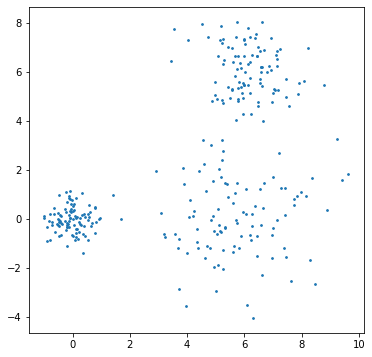In :
ax.scatter(x=centers.T, y=centers.T, s=80)
fig

Out: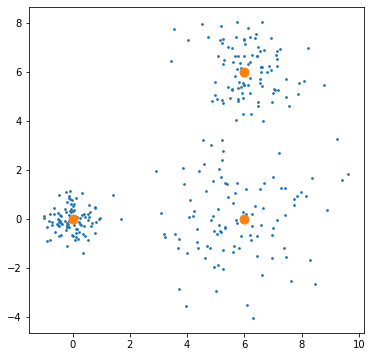And giving it some color so we can better differentiate the clusters:

In :
fig, ax = plt.subplots(figsize=(6,6))
ax.scatter(X.T, X.T, s=3, c=y);
ax.scatter(x=centers.T, y=centers.T, s=80, c=[0,1,2]);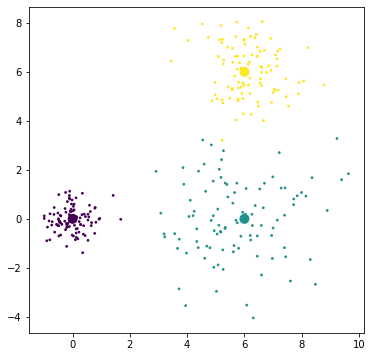As usual, let's split the data into training and validation datasets:

In :
from sklearn.model_selection import train_test_split
X_train, X_test, y_train, y_test = train_test_split(X, y, test_size=0.3, random_state=seed)


We can now build our clustering model and training using fit. K-means requires the number K of clusters as a parameter, so we will use our privileged knowledge and we will set K = 3:

In :
from sklearn.cluster import KMeans
kmeans = KMeans(n_clusters=3, random_state=seed).fit(X_train)


We can check the labels that the algorithm has assigned with the labels_ property:

In :
kmeans.labels_

Out:
array([2, 1, 0, 2, 2, 1, 1, 0, 1, 0, 1, 0, 1, 1, 2, 2, 0, 0, 1, 2, 0, 2,
1, 1, 1, 0, 0, 1, 2, 2, 0, 1, 1, 0, 0, 0, 1, 0, 2, 1, 0, 2, 1, 0,
1, 2, 0, 0, 0, 1, 0, 1, 1, 1, 1, 0, 1, 2, 2, 0, 2, 0, 0, 2, 1, 2,
2, 1, 2, 1, 0, 0, 1, 2, 1, 0, 0, 0, 1, 2, 1, 2, 0, 2, 1, 0, 1, 1,
2, 2, 1, 2, 0, 2, 0, 2, 1, 2, 0, 0, 2, 1, 0, 0, 0, 2, 0, 0, 2, 1,
1, 1, 0, 0, 2, 1, 0, 2, 1, 2, 1, 0, 0, 0, 1, 0, 0, 0, 1, 0, 1, 1,
0, 2, 1, 1, 2, 1, 0, 1, 2, 1, 1, 0, 2, 0, 1, 1, 2, 0, 2, 1, 2, 2,
2, 1, 2, 2, 1, 1, 2, 1, 0, 2, 0, 2, 1, 1, 0, 1, 0, 2, 0, 2, 2, 2,
2, 1, 1, 2, 2, 0, 2, 0, 2, 0, 0, 1, 0, 2, 0, 0, 0, 1, 1, 0, 0, 2,
1, 2, 1, 2, 0, 2, 1, 1, 2, 2, 1, 0], dtype=int32)

And the cluster centers obtained by k-means with cluster_centers_:

In :
kmeans.cluster_centers_.T

Out:
array([[ 6.14166207, -0.01398996,  5.74371096],
[ 5.93004311,  0.01725255, -0.24699213]])

If we compare the clustering obtained by k-means and the original cluster we can see it did a pretty good job (don't mind the cluster colors not matching from one plot to another, k-means re-assigns label values to each cluster when done training):

In :
fig, ax = plt.subplots(1,2,figsize=(14,6))
ax.scatter(X_train.T, X_train.T, s=3, c=y_train);
ax.scatter(x=centers.T, y=centers.T, s=80, c=[0,1,2]);
ax.set_title('Actual data')
ax.scatter(X_train.T, X_train.T, s=3, c=kmeans.labels_)
ax.scatter(x=kmeans.cluster_centers_.T, y=kmeans.cluster_centers_.T, s=80, c=[0,1,2])
ax.set_title('k-means clustering');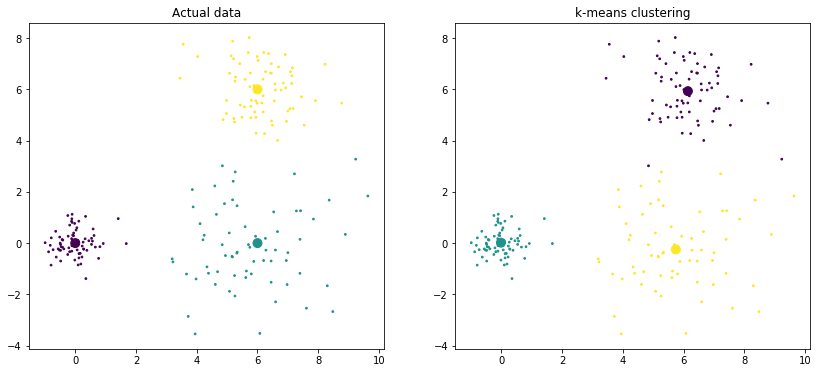Overlaying both plots, we can see the cluster centers have a pretty good match (actual centers in red):

In :
fig, ax = plt.subplots(figsize=(6,6))
ax.scatter(X_train.T, X_train.T, s=3, c=kmeans.labels_);
ax.scatter(x=kmeans.cluster_centers_.T, y=kmeans.cluster_centers_.T, s=80, c=[0,1,2])
ax.scatter(x=centers.T, y=centers.T, s=80, c=['red']);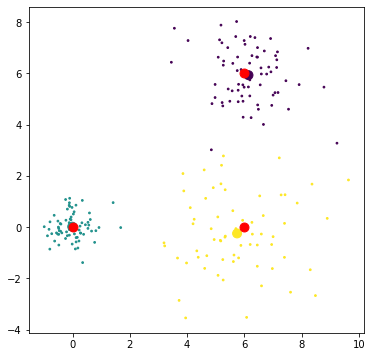Another way to evaluate the quality of a clustering is the homogeneity_score, which measures how many of the points in a cluster correspond to a single class:

In :
from sklearn.metrics import homogeneity_score
homogeneity_score(y_train, kmeans.labels_)

Out:
0.9603651641018862

The main drawback of homogeneity is that it only takes into account points within the same cluster belonging to the same class, so if all points in two different clusters belong to the same class the homogeneity score will be high even though there should be only one cluster.

One of the main issues with K-means is that you need to specify the number of clusters you want the algorithm to look for. As normally you do not know the amount of clusters beforehand tuning this hyperparameter can be tricky, specially for large values of k. For example, if we look for only two clusters in our dataset:

In :
kmeans2 = KMeans(n_clusters=2, random_state=seed).fit(X_train)
fig, ax = plt.subplots(figsize=(6,6))
ax.scatter(X_train.T, X_train.T, s=3, c=kmeans2.labels_);
ax.scatter(x=kmeans2.cluster_centers_.T, y=kmeans2.cluster_centers_.T, s=80, c=[0,1])
ax.scatter(x=centers.T, y=centers.T, s=80, c=['red']);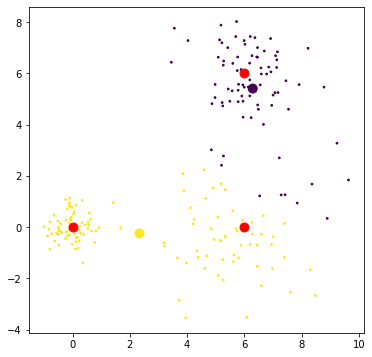We can see that k-means has aggregated two clusters into a single one, finding two clusters as we told it (original cluster centers in red). Checking the homogeneity score we can see its significantly lower:

In :
homogeneity_score(y_train, kmeans2.labels_)

Out:
0.47267561432978034

Alternatively, if we say K = 5:

In :
kmeans5 = KMeans(n_clusters=5, random_state=seed).fit(X_train)
fig, ax = plt.subplots(figsize=(6,6))
ax.scatter(X_train.T, X_train.T, s=3, c=kmeans5.labels_);
ax.scatter(x=kmeans5.cluster_centers_.T, y=kmeans5.cluster_centers_.T, s=80, c=[0,1,2,3,4])
ax.scatter(x=centers.T, y=centers.T, s=80, c=['red']);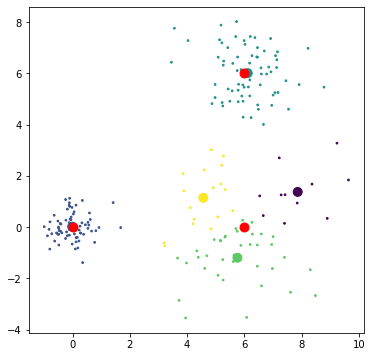We can see now that it has found two extra cluster that were not there in the first place. Calculating the homogeneity score:

In :
homogeneity_score(y_train, kmeans5.labels_)

Out:
0.9999999999999998

As we mentioned before, because it is only checking that point within each cluster belong to the same class, it has a score of effectively one. Taking it to an extreme case, if we made as many clusters as data points the homogeneity score would be 1 so you need to put some thought when using and interpreting the score.